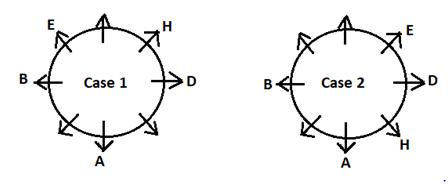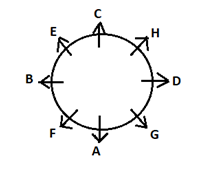# RRB Clerk Prelims Reasoning Ability Questions 2021 (Day-11)

Dear Aspirants, Our IBPS Guide team is providing new series of Reasoning Questions for RRB Clerk Prelims 2021 so the aspirants can practice it on a daily basis. These questions are framed by our skilled experts after understanding your needs thoroughly. Aspirants can practice these new series questions daily to familiarize with the exact exam pattern and make your preparation effective.

Start Quiz

Circular seating

Direction (1-5): Study the following information carefully and answer the questions given below:

Eight persons A, B, C, D, E, F, G, and H are sitting at a circular table facing outside the center, but not necessarily in the same order.

C doesn’t sit opposite to H. Two persons sit between A and E. H sits second to the right of E. Both B and D are sitting opposite to each other. Both H and B are not adjacent to each other. G sits immediate left of A.

1) How many persons are sitting between B and C when counted clockwise direction from B?

A.None

B.Four

C.Five

D.Three

E.One

2) Who among the following person is the immediate neighbor of both H and G?

A.D

B.F

C.A

D.B

E.None of these

3) Who among the following person sits third to the left of G?

A.H

B.D

C.E

D.C

E.None of these

4) Who among the following person sits opposite to C?

A.F

B.A

C.G

D.H

E.None of these

5) In a certain way D is related to A, and H is related to G, then who among the following is related to C?

A.D

B.B

C.F

D.A

E.None of these

Coding decoding

Direction (6-8): Study the following information carefully and answer the below questions.

In a certain code language

‘Cone Cube Pentagon Decagon’ means ‘lhm vtm ksm lpm’,

‘Rhombus Cube Heptagon Trapezoid’ means ‘zhm ytm lpm gtm’,

‘Heptagon Decagon Octagon Hexagon’ means ‘mhm lhm tvm gtm’,

‘Decagon Pentagon Octagon Ellipse’ means ‘lhm tvm vtm shm’

6) What does “shm” represent in a code language?

A.Ellipse

B.Pentagon

C.Octagon

D.Decagon

E.None of these

7) What is the code for ‘Hexagon Cone’ in the given language?

A.ksm mhm

B.lhm mhm

C.gtm ksm

D.tvm lhm

E.None of the above

8) Whatdoes ‘Zhm’ represent in the given language?

A.Rhombus

B.Trapezoid

C.Heptagon

D.Cube

E.Either a or b

Inequality

Direction (9-10): In each of the following questions, the relationship between different elements is shown in the statements followed by five conclusions. Find a true conclusion.

9) Statements:

G ≥ M > T = S > P;  V < T >N ≥ Q ;

Conclusions:

A.P ≥ M

B.G > Q

C.S < V

D.N > V

E.None of the above

10) Statements:

R ≥ K ≥ M = P ;   T < Z > S < P

Conclusions:

A.T < M

B.Z < K

C.R ≥ S

D.Z = R

E.None is true

Directions (1-5) :

As per the given statements, two persons sit between A and E. Here we have two possible cases i.e., Case 1 and Case 2.

H sits second to the right of E.

Both B and D are sitting opposite to each other.

Both H and B are not adjacent to each other.G sits immediate left of A.

Here Case 2 gets eliminated.

C doesn’t sit opposite to H.

Hence the final arrangement will be,Directions (6-8) :